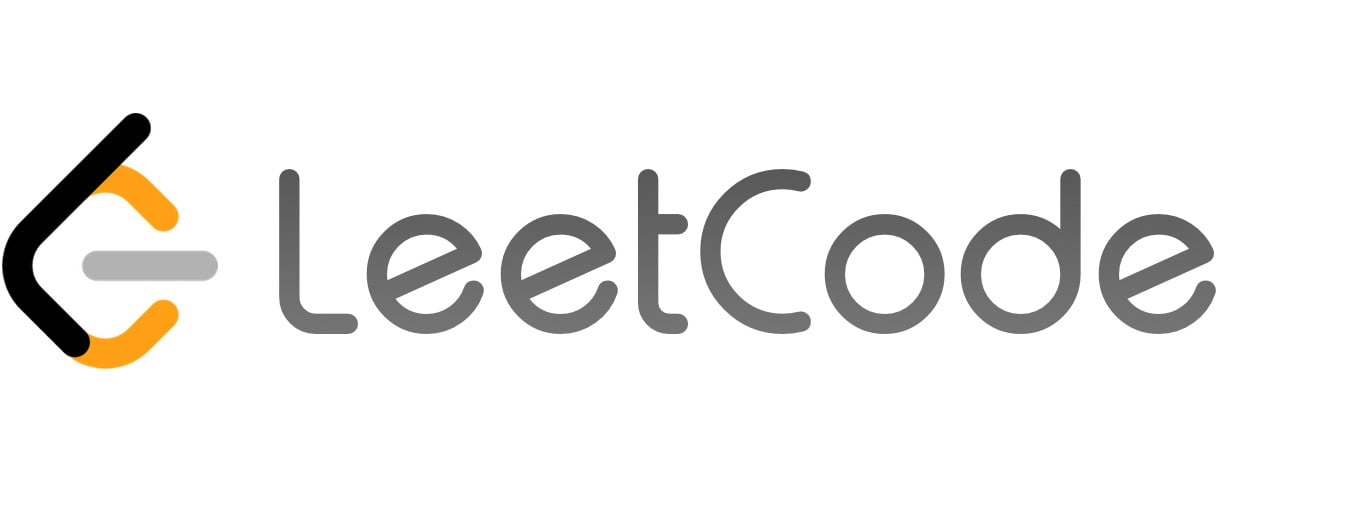LeetCode

## Editorial: LeetCode 1866 Number of Ways to Rearrange Sticks With K Sticks VisibleThinking process:

The brute force way to solve this is to find all permutations first, and then check if there are exactly `k` visible sticks. The time complexity would be O(N!*N) because we have N! permutations and we need O(N) time to check.

We can use recursive to solve this as well. We can start from left to right. We pick a stick first, if this stick is higher than the current highest stick, then we have one more visible stick. To do this, we need to know currently how many sticks are lower than the current highest one, and how many are higher than the current highest one, and the k.

As a result, we’ll have a recursive function like `int dfs(int lowers, int highers, int k)`. With this signature, even if we don’t consider how to transit between states, we already have 3 parameters, so the total states are O(N^3) already, which is too big because N <= 1000.

Now, this is the key point when interviwing with Google. Sometimes when we can’t solve this efficiently, try solve this backward. In this problem, when we go from left to right, we need to know how many sticks are lower than the current highest one and how many are higher. However, when we go from right to left, the only way to add a visible stick is to pick the highest one in the remaining sticks. All other sticks will not be visible. After knowing this, the code becomes simpler. We just use the common recursive function with a 2D array for the memorization.

Here is the code.

`````` 1class Solution {
2public:
3    int dp;
4    int rearrangeSticks(int n, int k, int mod = 1000000007) {
5        if (n == k) return 1;
6        if (k == 0) return 0;
7        if (dp[n][k] == 0)
8            dp[n][k]  = (1L * rearrangeSticks(n - 1, k - 1) + 1L * rearrangeSticks(n - 1, k) * (n - 1)) % mod;
9        return dp[n][k];
10    }
11};
``````